Ex 13.1 (Term 1)

Chapter 13 Class 11 Limits and Derivatives (Term 1 and Term 2)
Serial order wise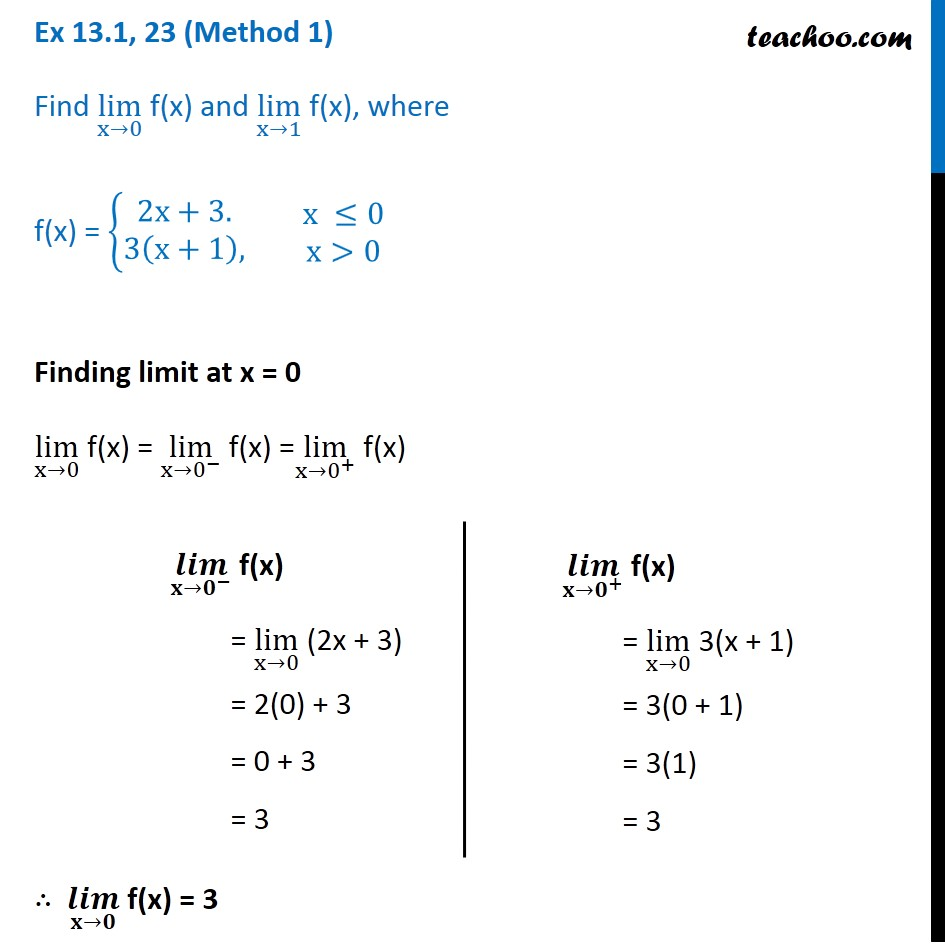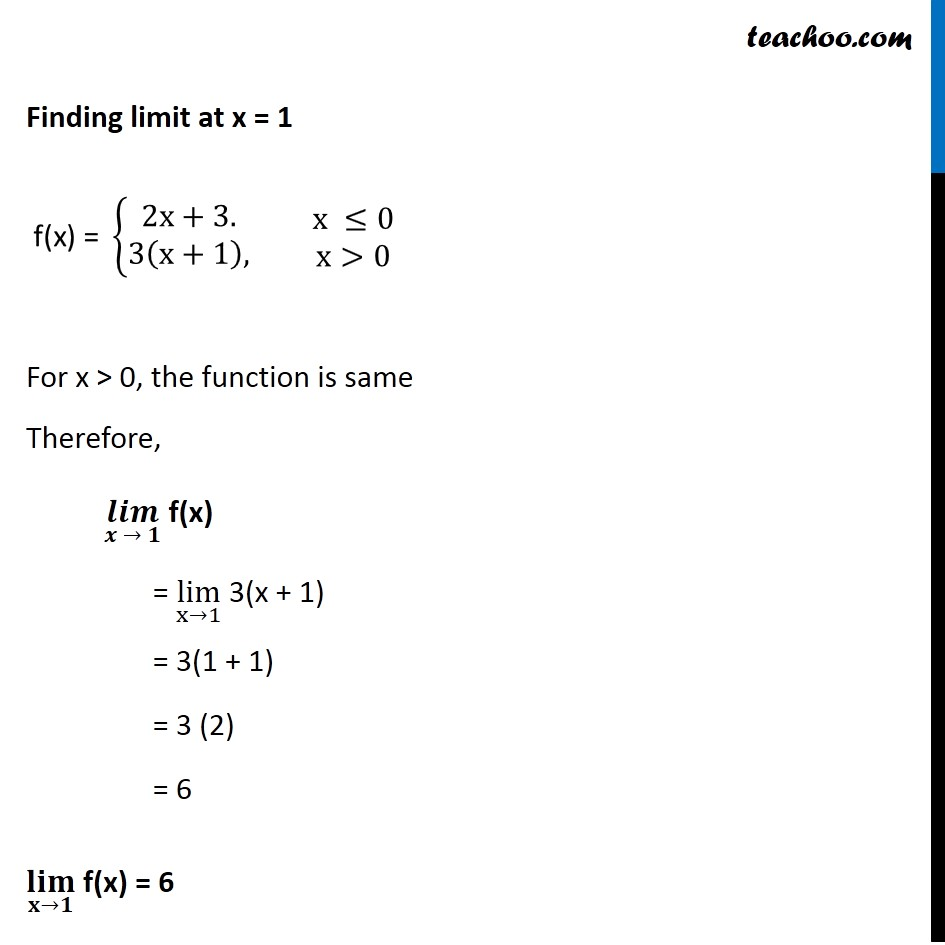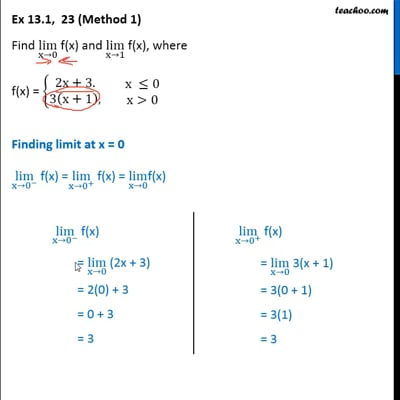This video is only available for Teachoo black users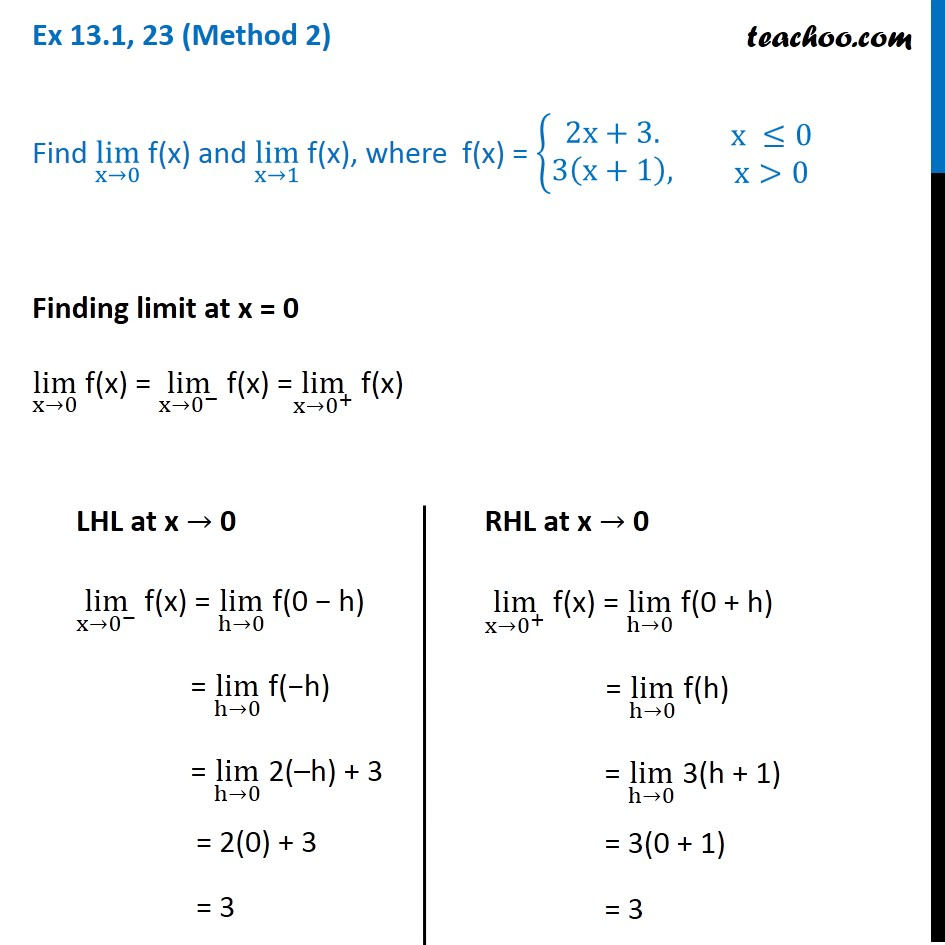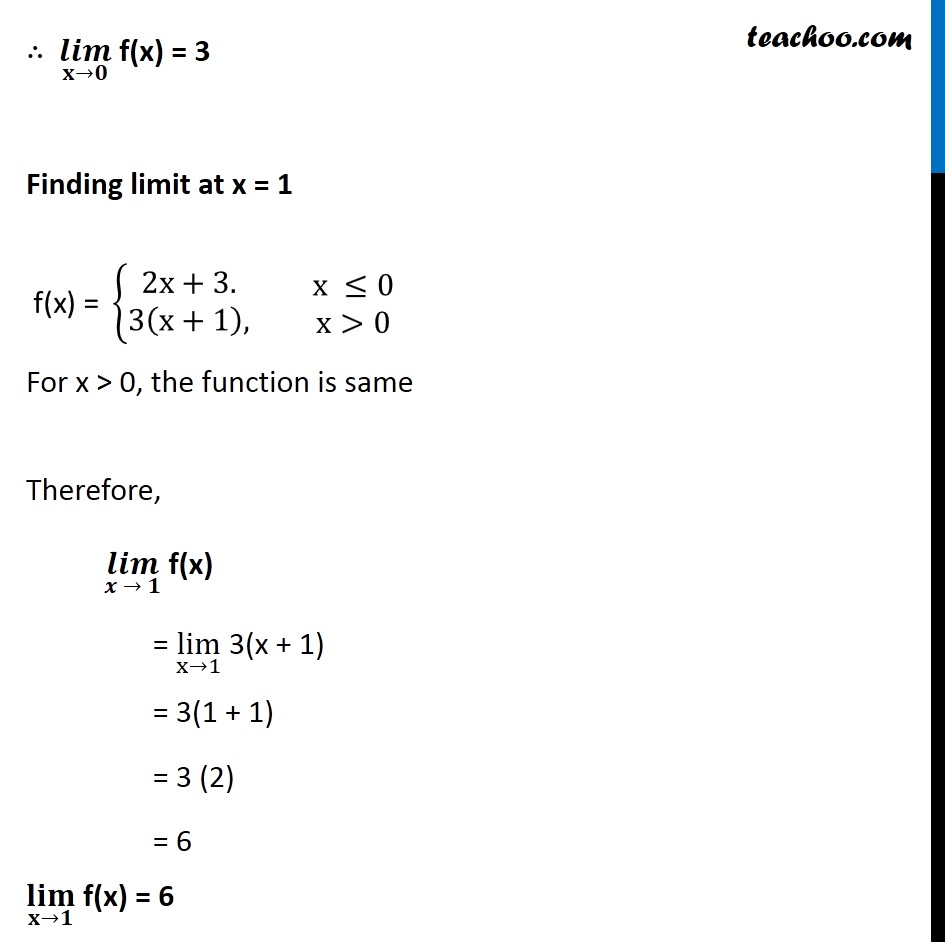### Transcript

Ex 13.1, 23 (Method 1) Find lim┬(x→0) f(x) and lim┬(x→1) f(x), where f(x) = {█(2x+3.@3(x+1),)┤ ■8(x ≤0@x>0) Finding limit at x = 0 lim┬(x→0) f(x) = lim┬(〖x→0〗^− ) f(x) =lim┬(〖x→0〗^+ ) f(x) ∴ (𝒍𝒊𝒎)┬(𝐱→𝟎) f(x) = 3 (𝒍𝒊𝒎)┬(〖𝐱→𝟎〗^− ) f(x) = lim┬(x→0) (2x + 3) = 2(0) + 3 = 0 + 3 = 3 (𝒍𝒊𝒎)┬(〖𝐱→𝟎〗^+ ) f(x) = lim┬(x→0) 3(x + 1) = 3(0 + 1) = 3(1) = 3 Finding limit at x = 1 "f(x) = " {█(2x+3.@3(x+1),)┤" " ■8(x ≤0@x>0) For x > 0, the function is same Therefore, (𝒍𝒊𝒎)┬(𝒙 → 𝟏) " f(x) " " = " lim┬(x→1) " 3(x + 1) " " = 3(1 + 1)" " = 3 (2)" " = 6" (𝐥𝐢𝐦)┬(𝐱→𝟏) f(x) = 6 Ex 13.1, 23 (Method 2) Find lim┬(x→0) f(x) and lim┬(x→1) f(x), where f(x) = {█(2x+3.@3(x+1),)┤ ■8(x ≤0@x>0) Finding limit at x = 0 lim┬(x→0) f(x) = lim┬(〖x→0〗^− ) f(x) =lim┬(〖x→0〗^+ ) f(x) LHL at x → 0 lim┬(x→0^− ) f(x) = lim┬(h→0) f(0 − h) = lim┬(h→0) f(−h) = lim┬(h→0) 2(–h) + 3 = 2(0) + 3 = 3 RHL at x → 0 lim┬(x→0^+ ) f(x) = lim┬(h→0) f(0 + h) = lim┬(h→0) f(h) = lim┬(h→0) 3(h + 1) = 3(0 + 1) = 3 ∴ (𝒍𝒊𝒎)┬(𝐱→𝟎) f(x) = 3 Finding limit at x = 1 "f(x) = " {█(2x+3.@3(x+1),)┤" " ■8(x ≤0@x>0) For x > 0, the function is same Therefore, (𝒍𝒊𝒎)┬(𝒙 → 𝟏) " f(x) " " = " lim┬(x→1) " 3(x + 1) " " = 3(1 + 1)" " = 3 (2)" " = 6" (𝐥𝐢𝐦)┬(𝐱→𝟏) f(x) = 6